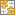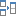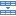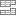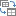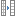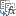# Excel: If cell contains formula examples

The tutorial provides a number of "Excel if contains" formula examples that show how to return something in another column if a target cell contains a required value, how to search with partial match and test multiple criteria with OR as well as AND logic.

One of the most common tasks in Excel is checking whether a cell contains a value of interest. What kind of value can that be? Just any text or number, specific text, or any value at all (not empty cell).

There exist several variations of "If cell contains" formula in Excel, depending on exactly what values you want to find. Generally, you will use the IF function to do a logical test, and return one value when the condition is met (cell contains) and/or another value when the condition is not met (cell does not contain). The below examples cover the most frequent scenarios.

## If cell contains any value, then

For starters, let's see how to find cells that contain anything at all: any text, number, or date. For this, we are going to use a simple IF formula that checks for non-blank cells.

IF(cell<>"", value_to_return, "")

For example, to return "Not blank" in column B if column A's cell in the same row contains any value, you enter the following formula in B2, and then double click the small green square in the lower-right corner to copy the formula down the column:

`=IF(A2<>"", "Not blank", "")`

The result will look similar to this:## If cell contains text, then

If you want to find only cells with text values ignoring numbers and dates, then use IF in combination with the ISTEXT function. Here's the generic formula to return some value in another cell if a target cell contains any text:

IF(ISTEXT(cell), value_to_return, "")

Supposing, you want to insert the word "yes" in column B if a cell in column A contains text. To have it done, put the following formula in B2:

`=IF(ISTEXT(A2), "Yes", "")`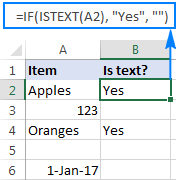## If cell contains number, then

In a similar fashion, you can identify cells with numeric values (numbers and dates). For this, use the IF function together with ISNUMBER:

IF(ISNUMBER(cell), value_to_return, "")

The following formula returns "yes" in column B if a corresponding cell in column A contains any number:

`=IF(ISNUMBER(A2), "Yes", "")`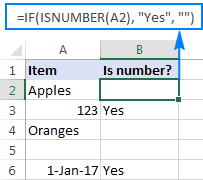## If cell contains specific text

Finding cells containing certain text (or numbers or dates) is easy. You write a regular IF formula that checks whether a target cell contains the desired text, and type the text to return in the value_if_true argument.

IF(cell="text", value_to_return, "")

For example, to find out if cell A2 contains "apples", use this formula:

`=IF(A2="apples", "Yes", "")`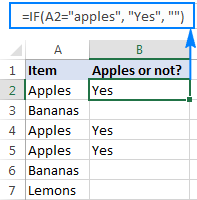### If cell does not contain specific text

If you are looking for the opposite result, i.e. return some value to another column if a target cell does not contain the specified text ("apples"), then do one of the following.

Supply an empty string ("") in the value_if_true argument, and text to return in the value_if_false argument:

`=IF(A2="apples", "", "Not apples")`

Or, put the "not equal to" operator in logical_test and text to return in value_if_true:

`=IF(A2<>"apples", "Not apples", "")`

Either way, the formula will produce this result: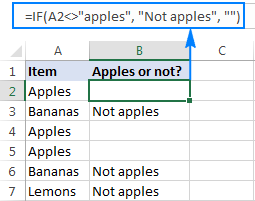### If cell contains text: case-sensitive formula

To force your formula to distinguish between uppercase and lowercase characters, use the EXACT function that checks whether two text strings are exactly equal, including the letter case:

`=IF(EXACT(A2,"APPLES"), "Yes", "")`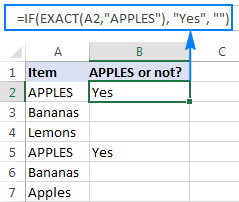You can also input the model text string in some cell (say in C1), fix the cell reference with the \$ sign (\$C\$1), and compare the target cell with that cell:

`=IF(EXACT(A2,\$C\$1), "Yes", "")`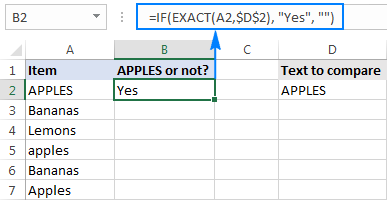## If cell contains specific text string (partial match)

We have finished with trivial tasks and move on to more challenging and interesting ones :) In this example, it takes three different functions to find out whether a given character or substring is part of the cell contents:

IF(ISNUMBER(SEARCH("text", cell)), value_to_return,"")

Working from the inside out, here is what the formula does:

• The SEARCH function searches for a text string, and if the string is found, returns the position of the first character, the #VALUE! error otherwise.
• The ISNUMBER function checks whether SEARCH succeeded or failed. If SEARCH has returned any number, ISNUMBER returns TRUE. If SEARCH results in an error, ISNUMBER returns FALSE.
• Finally, the IF function returns the specified value for cells that have TRUE in the logical test, an empty string ("") otherwise.

And now, let's see how this generic formula works in real-life worksheets.

### If cell contains certain text, put a value in another cell

Supposing you have a list of orders in column A and you want to find orders with a specific identifier, say "A-". The task can be accomplished with this formula:

`=IF(ISNUMBER(SEARCH("A-",A2)),"Valid","")`

Instead of hardcoding the string in the formula, you can input it in a separate cell (E1), the reference that cell in your formula:

`=IF(ISNUMBER(SEARCH(\$E\$1,A2)),"Valid","")`

For the formula to work correctly, be sure to lock the address of the cell containing the string with the \$ sign (absolute cell reference).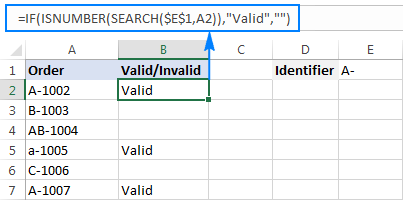### If cell contains specific text, copy it to another column

If you wish to copy the contents of the valid cells somewhere else, simply supply the address of the evaluated cell (A2) in the value_if_true argument:

`=IF(ISNUMBER(SEARCH(\$E\$1,A2)),A2,"")`

The screenshot below shows the results: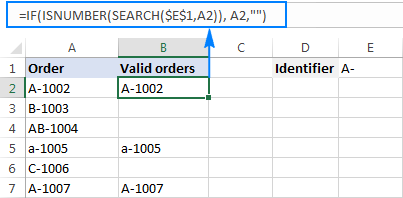### If cell contains specific text: case-sensitive formula

In both of the above examples, the formulas are case-insensitive. In situations when you work with case-sensitive data, use the FIND function instead of SEARCH to distinguish the character case.

For example, the following formula will identify only orders with the uppercase "A-" ignoring lowercase "a-".

`=IF(ISNUMBER(FIND("A-",A2)),"Valid","")`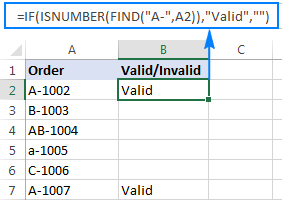## If cell contains one of many text strings (OR logic)

To identify cells containing at least one of many things you are searching for, use one of the following formulas.

### IF OR ISNUMBER SEARCH formula

The most obvious approach would be to check for each substring individually and have the OR function return TRUE in the logical test of the IF formula if at least one substring is found:

IF(OR(ISNUMBER(SEARCH("string1", cell)), ISNUMBER(SEARCH("string2", cell))), value_to_return, "")

Supposing you have a list of SKUs in column A and you want to find those that include either "dress" or "skirt". You can have it done by using this formula:

`=IF(OR(ISNUMBER(SEARCH("dress",A2)),ISNUMBER(SEARCH("skirt",A2))),"Valid ","")`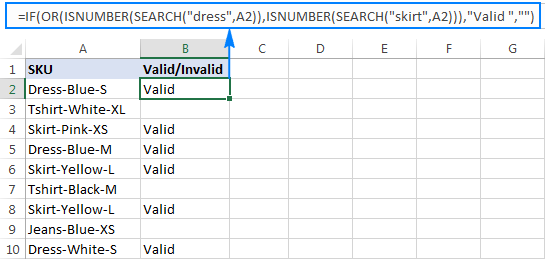The formula works pretty well for a couple of items, but it's certainly not the way to go if you want to check for many things. In this case, a better approach would be using the SUMPRODUCT function as shown in the next example.

### SUMPRODUCT ISNUMBER SEARCH formula

If you are dealing with multiple text strings, searching for each string individually would make your formula too long and difficult to read. A more elegant solution would be embedding the ISNUMBER SEARCH combination into the SUMPRODUCT function, and see if the result is greater than zero:

SUMPRODUCT(--ISNUMBER(SEARCH(strings, cell)))>0

For example, to find out if A2 contains any of the words input in cells D2:D4, use this formula:

`=SUMPRODUCT(--ISNUMBER(SEARCH(\$D\$2:\$D\$4,A2)))>0`

Alternatively, you can create a named range containing the strings to search for, or supply the words directly in the formula:

`=SUMPRODUCT(--ISNUMBER(SEARCH({"dress","skirt","jeans"},A2)))>0`

Either way, the result will be similar to this: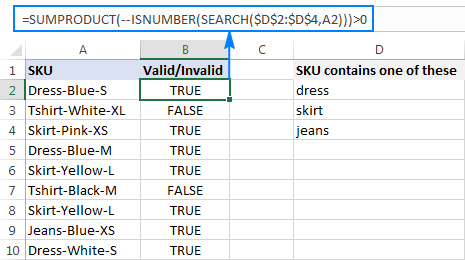To make the output more user-friendly, you can nest the above formula into the IF function and return your own text instead of the TRUE/FALSE values:

`=IF(SUMPRODUCT(--ISNUMBER(SEARCH(\$D\$2:\$D\$4,A2)))>0, "Valid", "")`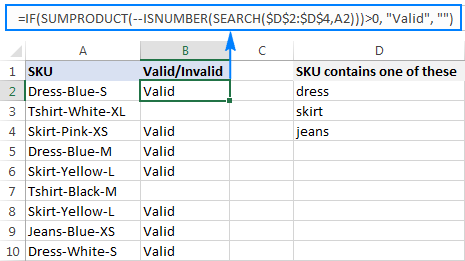#### How this formula works

At the core, you use ISNUMBER together with SEARCH as explained in the previous example. In this case, the search results are represented in the form of an array like {TRUE;FALSE;FALSE}. If a cell contains at least one of the specified substrings, there will be TRUE in the array. The double unary operator (--) coerces the TRUE / FALSE values to 1 and 0, respectively, and delivers an array like {1;0;0}. Finally, the SUMPRODUCT function adds up the numbers, and we pick out cells where the result is greater than zero.

## If cell contains several strings (AND logic)

In situations when you want to find cells containing all of the specified text strings, use the already familiar ISNUMBER SEARCH combination together with IF AND:

IF(AND(ISNUMBER(SEARCH("string1",cell)), ISNUMBER(SEARCH("string2",cell))), value_to_return,"")

For example, you can find SKUs containing both "dress" and "blue" with this formula:

`=IF(AND(ISNUMBER(SEARCH("dress",A2)),ISNUMBER(SEARCH("blue",A2))),"Valid ","")`

Or, you can type the strings in separate cells and reference those cells in your formula:

`=IF(AND(ISNUMBER(SEARCH(\$D\$2,A2)),ISNUMBER(SEARCH(\$E\$2,A2))),"Valid ","")`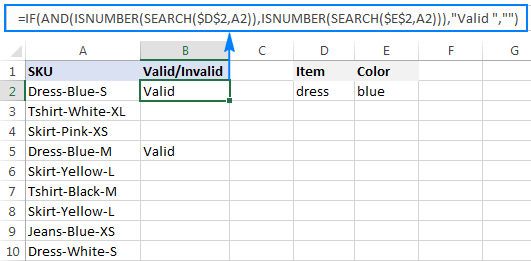As an alternative solution, you can count the occurrences of each string and check if each count is greater than zero:

`=IF(AND(COUNTIF(A2,"*dress*")>0,COUNTIF(A2,"*blue*")>0),"Valid","")`

The result will be exactly like shown in the screenshot above.

## How to return different results based on cell value

In case you want to compare each cell in the target column against another list of items and return a different value for each match, use one of the following approaches.

### Nested IFs

The logic of the nested IF formula is as simple as this: you use a separate IF function to test each condition, and return different values depending on the results of those tests.

IF(cell="lookup_text1", "return_text1", IF(cell="lookup_text2", "return_text2", IF(cell="lookup_text3", "return_text3", "")))

Supposing you have a list of items in column A and you want to have their abbreviations in column B. To have it done, use the following formula:

`=IF(A2="apple", "Ap", IF(A2="avocado", "Av", IF(A2="banana", "B", IF(A2="lemon", "L", ""))))`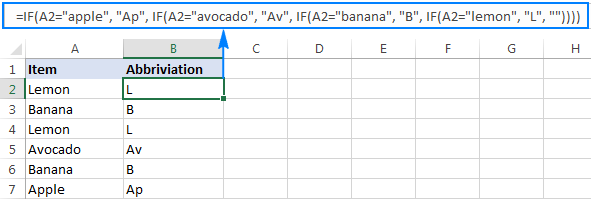For full details about nested IF's syntax and logic, please see Excel nested IF - multiple conditions in a single formula.

### Lookup formula

If you are looking for a more compact and better understandable formula, use the LOOKUP function with lookup and return values supplied as vertical array constants:

LOOKUP(cell, {"lookup_text1";"lookup_text2";"lookup_text3";…}, {"return_text1";"return_text2";"return_text3";…})

For accurate results, be sure to list the lookup values in alphabetical order, from A to Z.

`=LOOKUP(A2,{"apple";"avocado";"banana";"lemon"},{"Ap";"Av";"B";"L"})`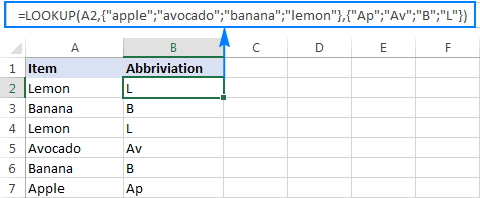Compared to nested IFs, the Lookup formula has one more advantage - it understands the wildcard characters and therefore can identify partial matches.

For example, if column A contains a few sorts of bananas, you can look up "*banana*" and have the same abbreviation ("B") returned for all such cells:

`=LOOKUP(A2,{"apple";"avocado";"*banana*";"lemon"},{"Ap";"Av";"B";"L"})`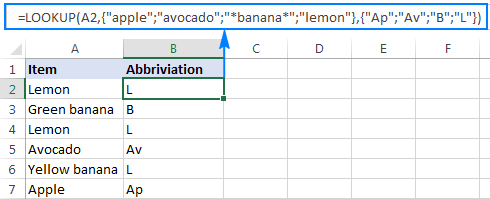### Vlookup formula

When working with a variable data set, it may be more convenient to input a list of matches in separate cells and retrieve them by using a Vlookup formula, e.g.:

`=VLOOKUP(A2, \$D\$2:\$E\$5, 2,FALSE )`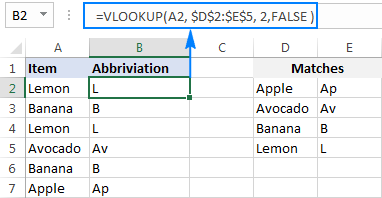This is how you check if a cell contains any value or specific text in Excel. To have a closer look at the formulas discussed in this tutorial, you are welcome to download our sample Excel If Contains workbook.

Next week, we are going to continue looking at Excel's If cell contains formulas and learn how to count or sum relevant cells, copy or remove entire rows containing those cells, and more. Please stay tuned!

### 42 responses to "Excel: If cell contains formula examples"

1.debbie says:

Hi, Mind appears simply, however i have tried several funtions to know avail,HELP

What i am trying to do is,
DATE OF APPOINTMENT DATE DUE

so todays date, then in date due, i want the date to show 90 days.

=IF(D3<(TODAY()+90),"<<<","")

help

regards Debbie

•Gennady Terekhov (Ablebits.com Team) says:

Hello,
For me to understand the problem better, please send me a small sample workbook with your source data and the result you expect to get to support@ablebits.com. Please don't worry if you have confidential information there, we never disclose the data we get from our customers and delete it as soon as the problem is resolved.

2.Robert says:

I scrolled thru your samples but did not find a match for what I'm really trying to accomplish

Column A contain a scroll option box
If Scroll option in cell A1 matches cell J3-J6 then B1=Good
If Scroll option in cell A1 matches cell J7-J12 then B1=Bad

Thanks

3.Tim C says:

Your suggestion on how to handle a cell that contains a specific string and do a partial match using combination of SEARCH, ISNUMBER and IF works like a charm! For example, my raw data input string in cell one is Apple, Ball, Cat, Dog and cell two is Apple, Dog. etc. etc. So I listed four separated columns in my modified data to store the results (1 or 0) if Apple, Ball, Cat or Dog is present or not in the string. I then reference the columns as tables and use a sumif to report on the respective tables. Works nicely. However I would like to pivot on the modified data and I want ONE field, not four. I would like one field showing table of Apple, Ball, Cat, Dog, Apple and Dog. One field name to be used by pivot table with six entries. How would I take the separated string results and put them back into a table so I can use a pivot table on that single table name?

4.Rajat Agarwal says:

Hi,

I have two data tables with multiple row and column data. in table 1, i have alphanumeric code and dates while in table 2 i have similar alphanumeric code. i wanted to search the table 2 for any part of the alphanumeric code from table 1 and on locating the same, fetching me the date againt the said code.

•Doug says:

Rajat:
Do you have sample data from each table you can post here? It's easier to try and help if I can see what you're working with.

5.Teddy says:

HI,

I Have a column, M, named "Qualifications". It contains different strings of Academic qualification data. But I just need to pick the specific qualification. E.g If the string reads " Masters of Education", I just need "Masters" If it reads "Certificate of Secondary Education", I need KCSE, If it reads "Bachelors degree in Medicine", I just need "Bachelors".

=LOOKUP(M2{"*Doctor*";"*Master*";"*Bachelor*";"*Diploma*";"*Secondary*";"*CSE*";"*EACE*";"*Primary*";"*CPE*";"*N/A*"} "PhD";"Masters";"Bachelors";"Diploma";"KCSE";"KCSE";"KCSE";"KCPE";"KCPE";"N/A"})

•Doug says:

Teddy:
Wildcards can be used in some functions, but not in others. If you need to use the * in the formula you'll need to use VLOOKUP or an INDEX/MATCH formula.
Here's how to write a nested IF statement for the samples you provided:

=IF(A74="Doctor","PHD", IF(A74="Master","Masters",IF(A74="Bachelor","Bachelors", IF(A74="Secondary","KCSE", IF(A74="Diploma","KCSE", IF(A74="CSE","KCSE", IF(A74="EACE","KCSE", IF(A74="Primary","CPE", IF(A74="N/A","N/A")))))))))

You can use this as the basis for a huge IF/OR statement, but it would get crazy long.
Read the VLOOKUP or INDEX/MATCH articles here on AbleBits and see if that helps.

6.Robert Davis says:

Good morning
What i am trying to achieve is to count the number of full stops in a cell and return a number based on that, i.e if i have 1 full stop then it will return a 1 and if it has 3 full stops then it will return a 3, it may very well be that there are up to 10 full stops in a cell. The formula from your examples i am using is =IF(ISNUMBER(SEARCH(\$C\$1,A1)),"1","")
This works great where C1 contains a full stop

7.basicdos says:

Help, please! I need a solution
Problem: B2 contains "IT2". In B7 I want to be shown that number which is found in B4, so B7 should contain "2". What is the correct logical formula?
So again: if a cell (B2) contains a number, show in another cell (B7) THAT number.

8.basicdos says:

"which is found in B4" sorry for typo, I mean B2

9.Tammy says:

Hi! I am need help building a formula. I have a spreadsheet to be filled in with data. Account codes are across the top. I trying to find a formula string that will recognize which cell across has any text, then return the account number at the heading of column. Not sure if that explanation makes sense. I'm sure there has to be a formula to avoid doing this manually.

10.Damon Martin says:

Is the following possible and if so what formula would I use to pull this off?
- Column A has rows of Summary data from problem tickets which will contain the problem ticket ID and other text.
- The problem ID will always be 15 characters in length
- The format of the Problem ID is USPM followed by the number for example USPM12345678911

Is there a formula that will look at for example cell A2 for *USPM* and return everything within the * * IN CELL b2? For example A2= USPM12345678911 the formula looks at A2 to see if it contains USPM and if it does it returns USPM and the next 11 charters to its right.

•Robert says:

=MID(A1,FIND("USPM",A1),19)

11.Lokie says:

Found this very helpful - thanks

12.Robert says:

Sorry, =MID(A1,FIND("USPM",A1),15)

The 15 or 19 should match the length of the text sting you want to return.

13.Kyle says:

I need a single formula that will say if there is a value in cell B1 then show 316, however if there is a value in C1 then show me 5000. I can get a formula that is on separate lines however, cannot get the formula in a single cell.
1 2
A Meter Number Amount
B 10HD00548 316
C 10HD00548 5000

Thank you.

14.Yakub says:

if a cell contains 12 Digits, then I want it to return specific text.
Example:- If A2 contains 12 Digits then, B2 should say "Good".
Can you help?

15.Martins says:

This is realy good if you have for example car models and you need to know what car it is
=IF(ISNUMBER(SEARCH("GO";A1));"MINI VAN";IF(ISNUMBER(SEARCH("YE";A1));"Bus";IF(ISNUMBER(SEARCH("L";A1));"Luksery line";"other")))))
and you can extend it as fares you need + it can have nr. of what year it was realised like you have L1 L2 L32 L13 L62 and its not important what nr. it is

16.ck says:

hi
I want to find if the cell contains NZ and then have the 3 numbers after the NZ as the result.
Example in cell D2 is DOL1003194 NZ101-05 in cell F2 I need the result to be 101
cell D3 is VOL10402 NZ102-077 in cell F3 I need the result to be 102
cell D4 is 51151317618 NZ112 in cell F4 I need the result to be 112

Be grateful for help

17.Graham Wilson says:

I have an order sheet containing, amongst other things, a description column and a value column. I need to put a comment such as "authorisation needed" in the description column when a value entered in the value column, in the corresponding line, is over £500.
I have tried conditional formatting and data validation but cannot get them to work together!
thinking I need some sort of "IF" formula but not well up on writing formulae.
any ideas would be appreciated - thanks

18.Dell says:

Hi, i need to find the amounts (column B) through finding the partial match (A2)to the long text (c2) and display the appropriate amount (column D).
LIST AMOUNTS LONG TEXT AMOUNT
HJA13784 ? abcd-HJA17561 09 082019 1,000,000
HJB02731 ? qwertyu-HJA13784 2019 08 2,500,000
HJA17561 ? plantferqfas sdsd ,HJA13784 3,000,000
SE18120347 ? asdfg sdg-SE10007894 4,000,000

19.Tim says:

Hi, i found this platform very useful in my daily work. Kudos to the guys managing this site.

I came across a problem that I am unable to find solutions or rather i might not know how to search the problem.

I have 2 workbooks(Report and Checklist)
In Report I have 2 columns, Item, Person
In Checklist I have Columns for Items. (5 Items, Apple, Grapes, Banana, HoneyDew and Orange), I have rows for Adam, John and Tom

In the Report Workbook. It shows this (the pipe symbol is to separate the columns)
Item | Person
Apple | Tom
Orange | JOHN
...

Expected outcome (In Checklist Workbook)
I want to match in the column of Adam and Apple to show as "Yes" and so forth.

20.Sahana says:

hi,
I came across an interesting problem need help to solve.
I have some text in Column A ( SKU ) and text to be searched in Column C ( Contains ), I need to search in SKU ( Column A) if any of the text listed in Contains ( Column C) need to insert value of Contains in Column B ( Print contains ) if none of the values in Contains ( Column C) is part of SKU ( Column A )then need to print No.
Expected result as below sample.
A B C
SKU Print contains Contains
--- ---------------- -----------
Dress-Blue-S dress dress
Tshirt-White-XL NO skrit
Skirt-Pink-XS skrit jeans
Skirt-Yellow-L NO
Tshirt-Black-M NO
Skrit-Yellow-L skrit
Jeans-Blue-XS jeans
Dress-White-S dress

21.Mario says:

Hello,
I have numbers on Column A1 that I need B1 to return with a name if the number matches
For example
A1 is 118 and B1 needs to be Chad
A1 is 132 and B1 needs to be Mike
A1 is 109 and B1 needs to be Tuan
A1 is 110 and B1 needs to be Kevin
A1 is 115 and B1 needs to be Carlos
A1 is 105 and B1 needs to be Mark
A1 is 107 and B1 needs to be Curtis

and so on, I have been fighting this all afternoon.

•ADEL says:

Use VLOOKUP formula

22.Roxanna says:

what would I use in the formula to lookup if a cell has text or number? (replacing the ISNUMBER) ISTEXT will not work as the cell can contain text or a number.
=IFERROR(IF(B17="","",IF(ISNUMBER(INDEX(T_E,MATCH(I_E,L_E,0),MATCH("ACT "&B17&" DT",L_H,0))),"R",CHAR(163))),"")

•MS says:

In your MATCH formula, what is the T_E , I_E and L_E? I believe that should be a range, but what range is it referring to?

23.Rhys says:

Hello
Is there a way of using the below formula, but rather than have it search for the specific text only within a cell, it can search a sentence containing "apple" or "banana" etc then return the value based on the sentence content? I need the formula to be able to search for multiple fruits and return the value in another cell depending on what fruit it found within the sentence.

For example, cell A1 contains the sentence, "Mr Smith ate an apple".
cell B1 should then return Apple. However, if cell A1 contained, "Mr Smith ate a banana", cell B2 should return "Banana".

=IF(A2="apple", "Ap", IF(A2="avocado", "Av", IF(A2="banana", "B", IF(A2="lemon", "L", ""))))

Hope this makes sense!

Thank you

•Svetlana Cheusheva (Ablebits.com Team) says:

Hi Rhys,

COUNTIF with wildcards in the criteria works a treat:

=IF(COUNTIF(A2, "*apple*")>0, "Ap", IF(COUNTIF(A2, "*avocado*")>0, "Av", IF(COUNTIF(A2, "*banana*")>0, "B", IF(COUNTIF(A2, "*lemon*")>0, "L", ""))))

•Rhys says:

Thanks so much! Worked perfectly.

24.Cherrypie Herrera says:

Hi , I have a list of products with the cost for each product is written in the adjacent cell . what I want is that when I type the name of the product in another worksheet , the value of the cost appears automatically . Can anybody please help me to find the exact equation for that ? thanks in advance

25.Becky says:

I have a budgeting document that reflects a few sub-budgets. I want there to be an overall balance column (G) and then 3 sub-budgets (I/J/K). I'd like a formula in I/J/K that states the following:

If column B includes the following partial text xxx (the budget indicator code), then subtract F from the row above it. (F is the amount spent).

The column B will include things like TS1, TS2, TS3 or HS1, HS2, HS3. (The TS and HS are the partial texts I want it to look for - with columns I and J being the TS and HS balance columns.)

26.Lynn Werner says:

HI, I need help with totaling up a number meeting a certain percent and the ablity to excluding any zeros. I can do the countif to obtain the percentage that achieves the required percent but I don't know how to exclude the zeros.
example: % achieving 80% w/o "0"
100
85
0
80
80
0

27.Jodi Andrews says:

-3.1441E-07x5
+ 9.9711E-05x4
- 1.2506E-02x3
+ 7.4496E-01x2
- 1.4013E+01x
+ 8.0642E+01
I am trying to use =IF(ISNUMBER(SEARCH("x",A1)), "LEFT(A1,FIND("x",A1)-1","A1") formula to look at each set of data and see it there is an "x" in the data string. If there is, I need to delete everything on the left of the "x", including the "x". However, if there is no 'x' I need the formula to sinply copy the data string as is, to the next cell. I may not have everthing just right but I now that the ISNUMBER(SEARCH is correct, I get TRUE, FALSE as I should. I have not been able to pair the formula with the rest, due to excel assuming that the "x" in the Left/Find statement is supposed to be a "*". Is there a way around this?
Thank you

28.Brendon says:

Hi, Please can someone assist with a formula that checks 2 separate columns and if the text is an exact match then it must return a a text from the separate column and also a value to the correct column.

29.JP says:

Hello,
I have a table in which I'm trying to match cities to markets. For example if I have a certain "City Name" in column G, I want a certain "Area name" in column J. I have tried all sorts of variations on this formula but am still a beginner and none of them seem to work.
Noting that there would me multiple cities in column G to match the same area in column J.
Any bit of help would be much appreciated. Thank you!

30.Rasit says:

Hi, upon some extensive research I couldn't find any answer to my problem. Here it is. Every month, I breakdown my transactions (from bank statements) according to their nature such as food, shopping and entertainment. So I prepared a key word mapping with natures as follows:

Column A - Column B

- Walmart - shopping
- Netlix - entertainment
- McDonalds - food

in two column in excel (list is pretty long, examples are simplified). Each transaction records includes many words and details of the transaction. What I want is, if a transaction includes the key word of **NETFLIX**, I need to bring word **ENTERTAINMENT** as nature next to the transaction column in excel. Example: if transaction is "*...June charge of **NETFLIX** obtained from credit card 12345...*" the key word **NETFLIX** is included in the transaction details, then bring **ENTERTAINMENT**. Had I kept my list short, I would have done it with =if(isnumber(search(... formula but my list is looong.

PS. Just extracting out the key word next to the transaction columnn would be fine, too. As the rest can be done by Vlookup.

31.Meghan says:

Hi there, hoping I didn't miss this explained above or in the comments but here's what i'm trying to figure out:
I have a list of titles in multiple rows of column I (Ex. I2 contains Associate, Manager, Senior Manager, Vice President. I3 contains Associate, Senior Manager, Vice President).
I am using the following formula to separate each title into separate columns for each Row, if it is listed in "I" : =IF(ISNUMBER(SEARCH("Associate",I2)),"Associate") but I'm finding that when using the formula for Manager it is giving me a false positive because "Senior Manager" contains the word "Manager" (is should result in "FALSE").

Basically, is there a way to add an exclusion for the word "senior" in the formula?

Thanks!

•Alexander Trifuntov (Ablebits.com Team) says:

Hello Meghan!
If I understand your task correctly, the following formula should work for you:

=IF(ISNUMBER(SEARCH("Manager",I3)), IF(ISNUMBER(SEARCH("Senior Manager",I3)),FALSE,"Associate"))

I hope this will help

32.Kapil Kaushik says:

my column A contains the following formula:
=INDIRECT("'"&\$D\$2&"'!"&Parameters!N\$2&\$C22)
The formula gets values from another tab based on certain parameters.
Using conditional formatting I want to make sure the indirect formula exists in all the cells and no one has tempered with the formula. Is there a way to conditional format the column A to highlight cells using the Indirect formula?
Thanks

KK

•Alexander Trifuntov (Ablebits.com Team) says:

Hello!
It is very difficult to understand a formula that contains unique references to your workbook worksheets. For the same reason, I cannot check her work.
I recommend using the FORMULATEXT function. It will extract the formula from the desired cell and write it down as text. You can compare this text with your formula if you also write it as text.

Ultimate Suite for Microsoft Excel